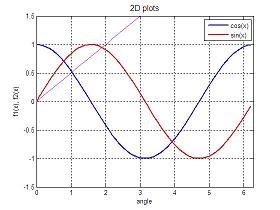2D Plots - Fancy Graphic Tool

Matlab includes fancy tools for visualization. Basic 2D plots, good 3D graphics, and even animation possibilities are available in an easy environment.

The most basic and useful command for producing simple 2D plots is:

plot(xvalues, yvalues, 'style')
• xvalues is the value of the horizontal points to be plotted.
• yvalues is the value of the function to be plotted.
• xvalues and yvalues have to have the same length.
• 'style' is an optional argument that specifies the color, line style and point-marker style.

Examples:

 plot(x,y) plots y vs x with a solid line plot(x,y,'-.') plots y vs x with a dash-dot line plot(x) plots the elements of x against their own index plot(x,y,'r--') plots y vs x with a red dashed line plot(a,b,'k+') plots b vs a with black plus signs

You may annotate your plots with 'xlabel', 'ylabel' and 'title'. Other useful functions are 'legend', 'axis', 'grid' and 'hold'.

Here's an example that integrates all of the above functions.

% Clears variables, command window, and closes all figures
clear, clc,close all

% Defines value of x and two functions
x = 0: .1 : 2*pi;
y1 = cos(x);
y2 = sin(x);

% Plots two functions with different style, and wider lines
plot(x,y1,'b', x, y2, 'r-.', 'linewidth', 2)

% Activates the grid
grid on

% Defines limits for x and y axes, and sets title, labels and legends
axis([0 2*pi -1.5 1.5])
title('2D plots', 'fontsize', 12)
xlabel('angle')
ylabel('f1(x), f2(x)')
legend('cos(x)', 'sin(x)')

% Keeps figure on screen, in order to add a third function
hold on

% Defines another function
y3 = 0.5 * x;

% Plots over the previous figure
plot(x, y3, 'm')

And the result is: